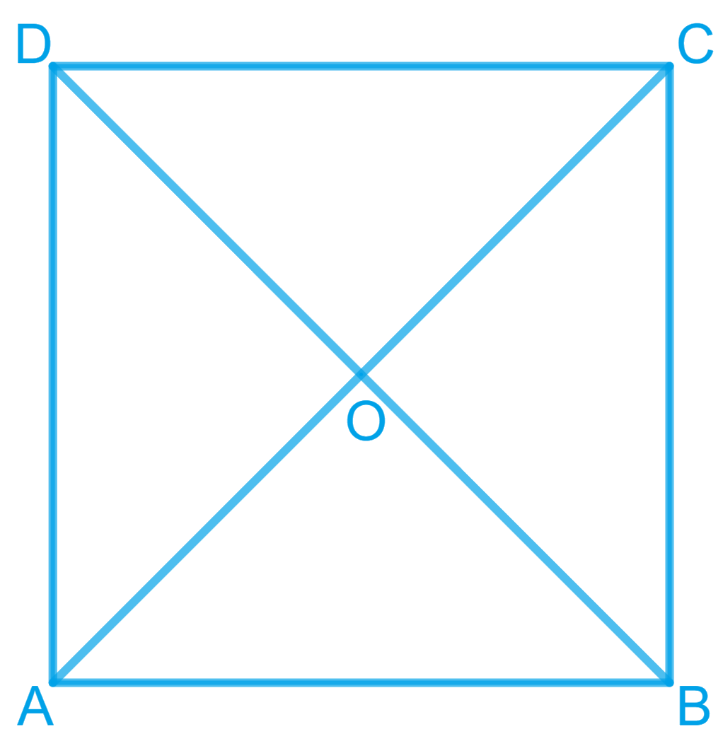# Ex.8.1 Q4 Quadrilaterals Solution - NCERT Maths Class 9

Go back to  'Ex.8.1'

## Question

Show that the diagonals of a square are equal and bisect each other at right angles.

Video Solution
Ex 8.1 | Question 4

## Text Solution

What is known?

What is unknown?

How we can show that diagonals of a square are equal and bisect each other at right angles.

Reasoning:

By showing two triangles congruent consist diagonals and then we can say corresponding parts of congruent triangles are equal.

Steps:Let $$ABCD$$ be a square. Let the diagonals $$AC$$ and $$BD$$ intersect each other at a point $$O$$. To prove that the diagonals of a square are equal and bisect each other at right angles, we have to prove,

\begin{align}&\mathrm{AC}=\mathrm{BD}, \mathrm{OA}=\mathrm{OC}, \mathrm{OB}=\mathrm{OD},\end{align} and \begin{align} \angle \mathrm{AOB}=90^{\circ} \end{align}

In $$\triangle \mathrm{ABC} \text { and } \Delta \mathrm{DCB}$$

\begin{align} AB&={DC}\left[\begin{array}{}\text {Sides of a square are}\\ \text{equal to each other}\end{array}\right]\\{\angle {ABC}}&\!=\!\angle {DCB} \left[\!\begin{array}{}\text{All interior angles }\\ \text{ are of }90^\circ \end{array}\!\right]\\ {{BC}}&={{CB} \quad \text{(Common side) }} \\ ∴ \Delta {ABC} &\cong \Delta {DCB} \left[\begin{array}{}\text{By SAS }\\ \text{congruency}\end{array}\right] \\ {∴ \; {AC}}&={{DB} \quad\text{(By CPCT )}}\end{align}

Hence, the diagonals of a square are equal in length.

In $$\Delta {{AOB \;\rm and }}\;\Delta {{COD ,}}$$

\begin{align}\angle {{AOB}} &= \angle {{COD}}\\\text{(Vertically }&\text {opposite angles)}\\\\\angle {{ABO}} &= \angle {{CDO}}\\\text{(Alternate}&\text { interior angles)}\\\\{{AB}} &= {{CD}}\\\text{( Sides of a square}&\text { are always equal)}\\\\\therefore \Delta {{AOB}}&\cong \Delta {{COD }}\\ \text{(By AAS}&\text { congruence rule)}\\\\ \therefore {{AO}} &= {{CO \;\rm and \;OB}} = {{OD}}\\ &\text{(By CPCT)}\end{align}

Hence, the diagonals of a square bisect each other.

In \begin{align} \Delta {AOB} \end{align} and \begin{align} \Delta {COB}, \end{align}

As we had proved that diagonals bisect each other, therefore,

\begin{align} {{AO}}&={{CO}} \\ {{AB}}&={CB}\\ \text{( Sides of a}&\text { square are equal )} \\ \\{{BO}}&={{BO} \,\quad \text{( Common )}}\\{∴\Delta {AOB} }&\cong \Delta {COB}\\ \text{(By SSS}&\text { congruency )} \\\\ \therefore {\angle {AOB}}&={\angle {COB}\,\quad \text{(By CPCT)}}\end{align}

However,

\begin{align}\angle {AOB}+\angle {COB}&=180^{\circ} \\2 \angle {AOB} &=180^{\circ} \\ \angle {AOB}&=90^{\circ}\end{align}

Hence, the diagonals of a square bisect each other at right angles.

Video Solution